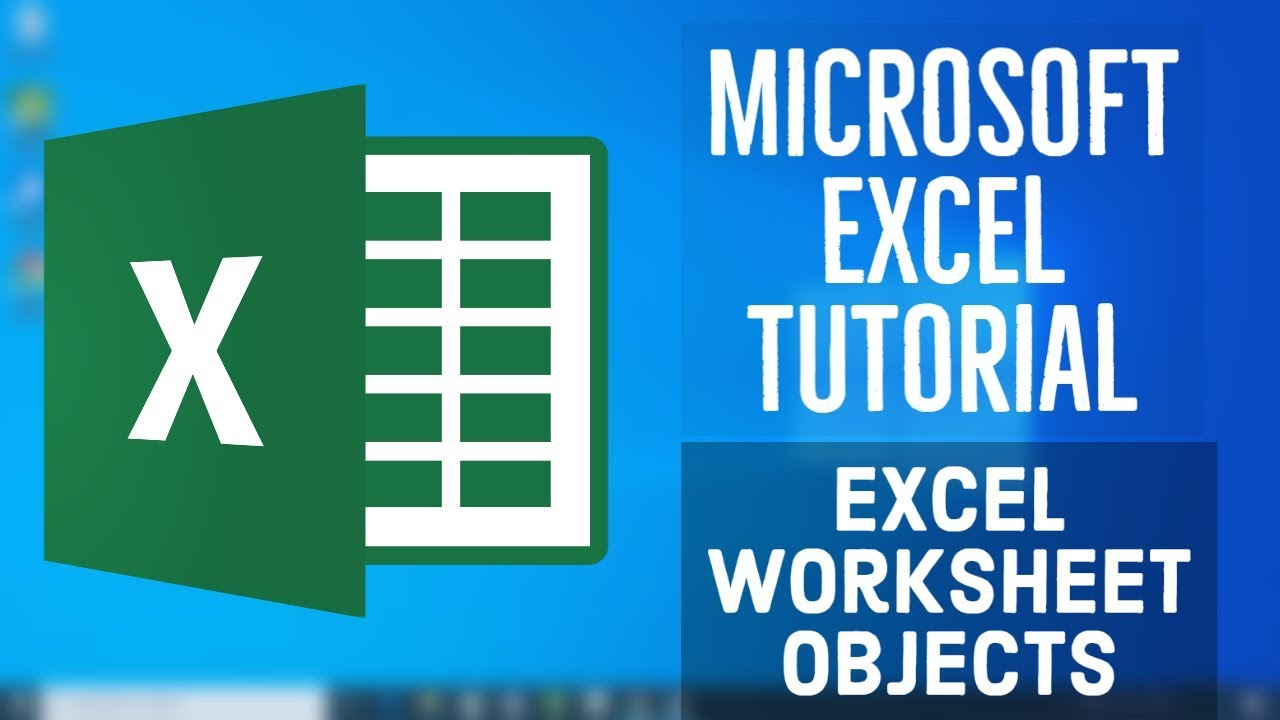# Microsoft Excel Tutorial - Objects In Ms Excel- Excel Worksheet ObjectsIn this MS Excel tutorials, video we are going to see the differnce between tables and cell ranges in MS Excel. Also we are going to see that how can we create a Table from data given to us and what all changes we can apply to our tables.

In this MS Excel tutorials, video we are going to see the differnce between tables and cell ranges in MS Excel. Also we are going to see that how can we create a Table from data given to us and what all changes we can apply to our tables.

Welcome to the The Beginner's Guide course to Excel. This course enables you to Learn MS Excel in simple and easy steps. In this Microsoft Excel Basics Tutorial series we will start from the basics and gradually move towards the Expert level in Microsoft Excel. This MS Excel course provides the Beginners to Intermediate Excel Skills, Tips, and Tricks. In this course we will learn how to Enter and edit Excel data, Format numbers, fonts and alignment, Make simple pivot tables and charts, Create simple Excel formulas, How to Use Excel Functions IF and VLOOKUP. Learn common Excel functions used in any Office, How to Create dynamic reports, Build Excel formulas to analyze date, text fields, values and arrays and much more advanced stuff.

In this video we will see the Overview of formulas in Excel. We will see Basic Excel formulas & functions with examples .

## Microsoft Excel Tutorial - Slicers on Charts in MS Excel.

In this MS Excel tutorials, video we are going to apply the slicers in charts in MS Excel. However, there is no direct method of doing so, that is why we are going to use a simple trick over here. Also we are going to see that how can we connect same slicer with multiple charts in MS Excel. ​

## Data Validation in Excel

What is Data Validation in Excel. Data validation is a feature in Excel used to control what a user can enter into a cell. Learn more about it with Examples.

## Microsoft Excel Tutorial - Alt Key Shortcuts in MS Excel.

In this MS Excel tutorials video we are going to look at the shortcuts of Alt keys in MS Excel. The advantage we get here is constant visual aid is available, which allows beginners to use these shortcuts very easily.

## Microsoft Excel Tutorial - Table vs Cell Range in MS Excel

We are going to see the differnce between tables and cell ranges in MS Excel. Also we are going to see that how can we crea...

## Microsoft Excel Tutorial - Summary Report in MS Excel

In this MS Excel tutorials video, we are going to see how to create Summary Report in MS Excel. Also, in this video we are going to see that how different functions like Sum, Count and Average can be used over the data in Pivot Tables.